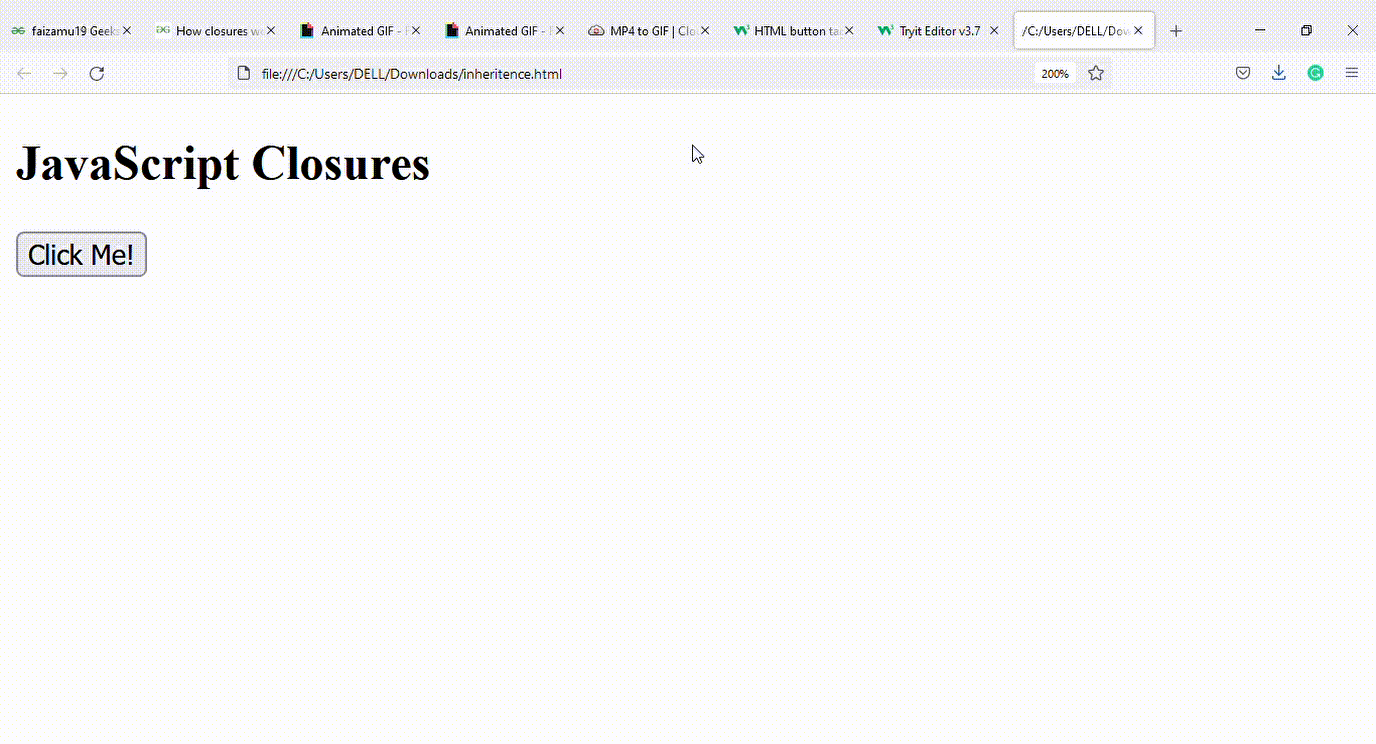Open in App
Not now

# How closure works in JavaScript ?

• Last Updated : 23 Nov, 2021

In this article, we will discuss about the closures working JavaScript. Let us first understand what exactly closures are and basic details which are associated with closures in JavaScript.

A Closure is a combination of a function enclosed with references to its surrounding state (the lexical environment). In JavaScript, closures are created every time a function is created at run time. In other words, a closure is just a fancy name for a function that remembers the external things used inside it.

Let us look at some examples to understand how closures actually work in JavaScript.

Example 1:

• In this example, we will be declaring a closure which would eventually access an outer variable balance from the outer function.
• After using the outer variable in inner most function, that particular closure will help us to deduct 100 from it, each time whenever that outer function is called.

## HTML

 ```<``html``>`` ` `<``body``>``    ``<``h2``>JavaScript Closures``    ``<``button` `type``=``"button"` `onclick``=``"initaccount()"``>``          ``Click Me!``        ````    ``<``p` `id``=``"demo"``>``    ``<``script``>``    ``function initaccount() {``        ``var balance = 1000;`` ` `        ``function currentbalance() {``            ``balance = balance - 100;``            ``alert(balance);``        ``}``        ``currentbalance();``    ``}``    `````` ` ``

Output:Explanation: In the above example, currentbalance() can access outer variable balance hence balance is deducted by 100 each time initaccount() method is called.

Example 2: Closures can be nested as well as in below example. Here in the example both outerfunction() and innerfunction() has access to counter variable , hence on calling Counter() both outerfunction() and innerfunction() increments the value of counter. In this context, we can say that closures have access to all outer function scopes.

## HTML

 ```<``html``>`` ` `<``body``>``    ``<``h2``>JavaScript Closures``    ``<``button` `type``=``"button"` `onclick``=``" Counter()"``>``        ``Click Me!``    ````    ``<``p` `id``=``"demo1"``>``    ``<``p` `id``=``"demo2"``>``    ``<``script``>``    ``function Counter() {``        ``var counter = 0;`` ` `        ``function outerfunction() {``            ``counter += 1;``            ``document.getElementById("demo1").innerHTML``                ``= "outercounter = " + counter +``                ``"from outerfunction " ;`` ` `            ``function innerfunction() {``                ``counter += 1;``                ``document.getElementById("demo2").innerHTML``                 ``= " innercounter = " + counter + ``                   ``"from innerfunction ";``            ``};``            ``innerfunction();``        ``};``        ``outerfunction();``    ``};``    `````` ` ``

Output:My Personal Notes arrow_drop_up